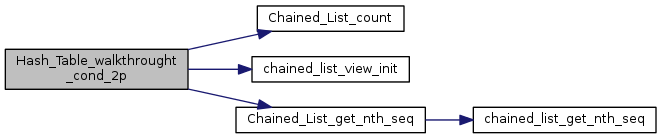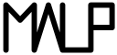Multi-ApplicationOnlineProfiling  2.1
Walkers

Functions that executes functions on elements. More...

## Functions

void Hash_Table_walkthrought (struct Hash_Table *ht, void(*action)(void *))
Executes a function on every stored elements. More...

void Hash_Table_walkthrought_2p (struct Hash_Table *ht, void *second_arg, void(*action)(void *, void *))
Executes a function on every stored elements. More...

void Hash_Table_walkthrought_3p (struct Hash_Table *ht, void *second_arg, void *third_arg, void(*action)(void *, void *, void *))
Executes a function on every stored elements. More...

void Hash_Table_walkthrought_cond (struct Hash_Table *ht, void(*action)(void *), int(*test_func)(void *, void *), void *test_value)
Executes a function on every stored elements that satisfy a condition. More...

void Hash_Table_walkthrought_cond_2p (struct Hash_Table *ht, void *second_arg, void(*action)(void *, void *), int(*test_func)(void *, void *), void *test_value)
Executes a function on every stored elements that satisfy a condition. More...

## Detailed Description

Functions that executes functions on elements.

## Function Documentation

 void Hash_Table_walkthrought ( struct Hash_Table * ht, void(*)(void *) action )

Executes a function on every stored elements.

Parameters
 ht the hashtable where to walk through action the function to execute on all elements (takes an element)

Definition at line 350 of file Hash_Table.c.

Here is the call graph for this function: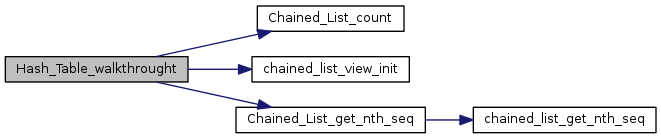Here is the caller graph for this function: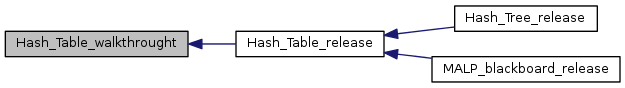void Hash_Table_walkthrought_2p ( struct Hash_Table * ht, void * second_arg, void(*)(void *, void *) action )

Executes a function on every stored elements.

Parameters
 ht the hashtable where to walk through second_arg an other argument to pass to action action the function to execute on all elements (takes an element and an other argument)

Definition at line 385 of file Hash_Table.c.

Here is the call graph for this function: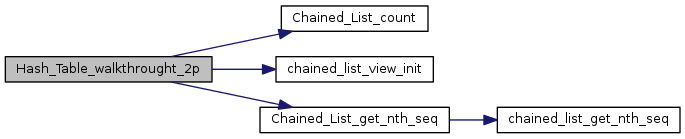Here is the caller graph for this function: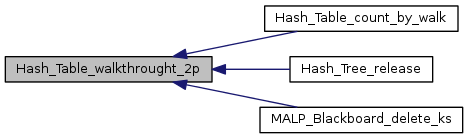void Hash_Table_walkthrought_3p ( struct Hash_Table * ht, void * second_arg, void * third_arg, void(*)(void *, void *, void *) action )

Executes a function on every stored elements.

Parameters
 ht the hashtable where to walk through second_arg an other argument to pass to action third_arg an other argument to pass to action action the function to execute on all elements (takes an element and two other arguments)

Definition at line 435 of file Hash_Table.c.

Here is the call graph for this function: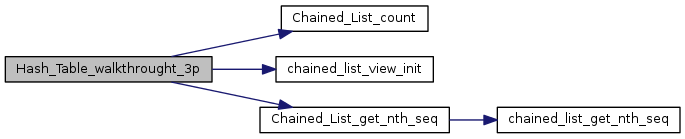Here is the caller graph for this function: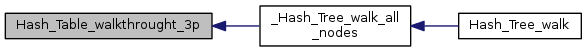void Hash_Table_walkthrought_cond ( struct Hash_Table * ht, void(*)(void *) action, int(*)(void *, void *) test_func, void * test_value )

Executes a function on every stored elements that satisfy a condition.

Parameters
 ht the hashtable where to walk through action what to execute on satisfying elements (takes an element) test_func condition function. takes an element and the test_value. return 1 if success test_value a test value to give to test_func

Definition at line 232 of file Hash_Table.c.

Here is the call graph for this function: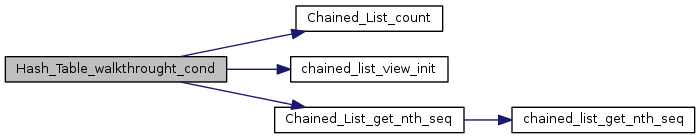void Hash_Table_walkthrought_cond_2p ( struct Hash_Table * ht, void * second_arg, void(*)(void *, void *) action, int(*)(void *, void *) test_func, void * test_value )

Executes a function on every stored elements that satisfy a condition.

Parameters
 ht the hashtable where to walk through second_arg the second argument to pass to action action what to execute on satisfying elements (takes an element and an other argument) test_func condition function. takes an element and the test_value. return 1 if success test_value a test value to give to test_func

Definition at line 282 of file Hash_Table.c.

Here is the call graph for this function: